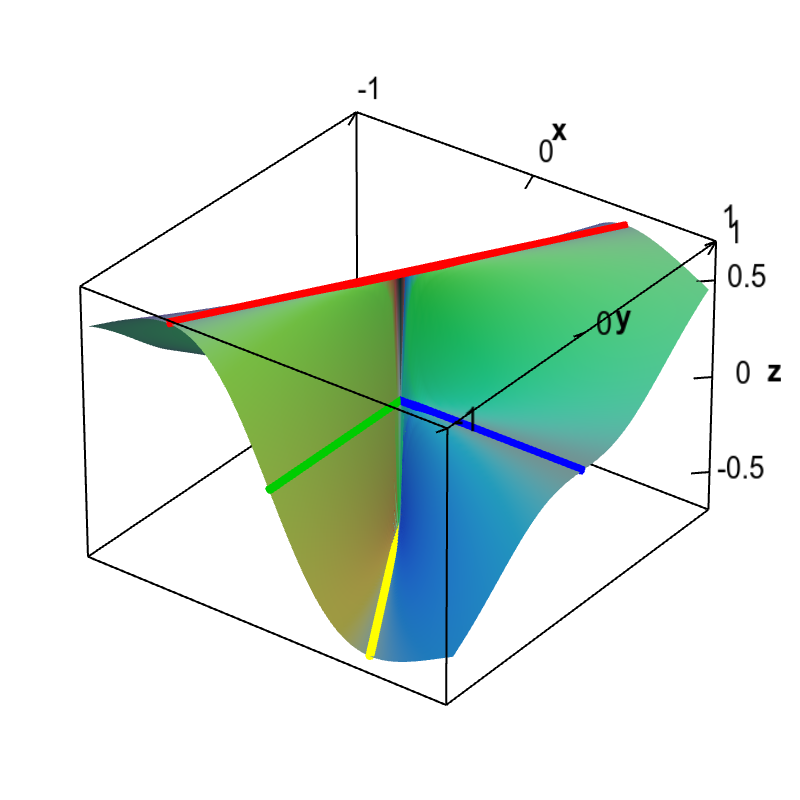# Math Insight

### Applet: Lines demonstrating the discontinuity of the partial x derivative of a non-differentiable functionThis graph shows the partial derivative with respect to $x$ of a function $f(x,y)$ that is not differentiable at the origin. The discontinuity of $\pdiff{f}{x}(x,y)$ is demonstrated by overlaying lines along which $\pdiff{f}{x}$ is constant. Along the blue line of the $x$-axis and the green line of the $y$ axis, $\pdiff{f}{x}$ is zero. However, along the line $y=x\sqrt{3}$ (in red), $\pdiff{f}{x}=3\sqrt{3}/8 \approx 0.65$, and along the line $y=-x\sqrt{3}$ (in yellow), $\pdiff{f}{x}=-3\sqrt{3}/8 \approx -0.65$. Since within any neighborhood of the origin, $\pdiff{f}{x}(x,y)$ takes on these different values, the partial derivative $\pdiff{f}{x}(x,y)$ is discontinuous at the origin.

This function is \begin{align*} f(x,y) = \begin{cases} \displaystyle \frac{x^2y}{x^2+y^2} & \text{if } (x,y) \ne (0,0)\\ 0 & \text{if } (x,y) = (0.0). \end{cases} \end{align*} Its partial derivative with respect to $x$ is \begin{align*} \pdiff{f}{x}(x,y) &= \begin{cases} \displaystyle \frac{2 x y}{x^2+y^2}-\frac{2 x^3 y}{\left(x^2+y^2\right)^2} \ & \text{if } (x,y) \ne (0,0)\\ 0 & \text{if } (x,y) = (0.0). \end{cases} \end{align*} (One must use the limit definition to calculate the partial derivative at the origin.)

Plugging in $y=x\sqrt{3}$ gives $$\diff{f}{x}(x,x\sqrt{3})=\frac{3\sqrt{3}}{8}$$ and plugging in $y=-x\sqrt{3}$ gives $$\diff{f}{x}(x,-x\sqrt{3})=\frac{-3\sqrt{3}}{8}$$ as long as $x \ne 0$. Since both these values occur arbitrarily near the origin, $\pdiff{f}{x}(x,y)$ is discontinuous at the origin.